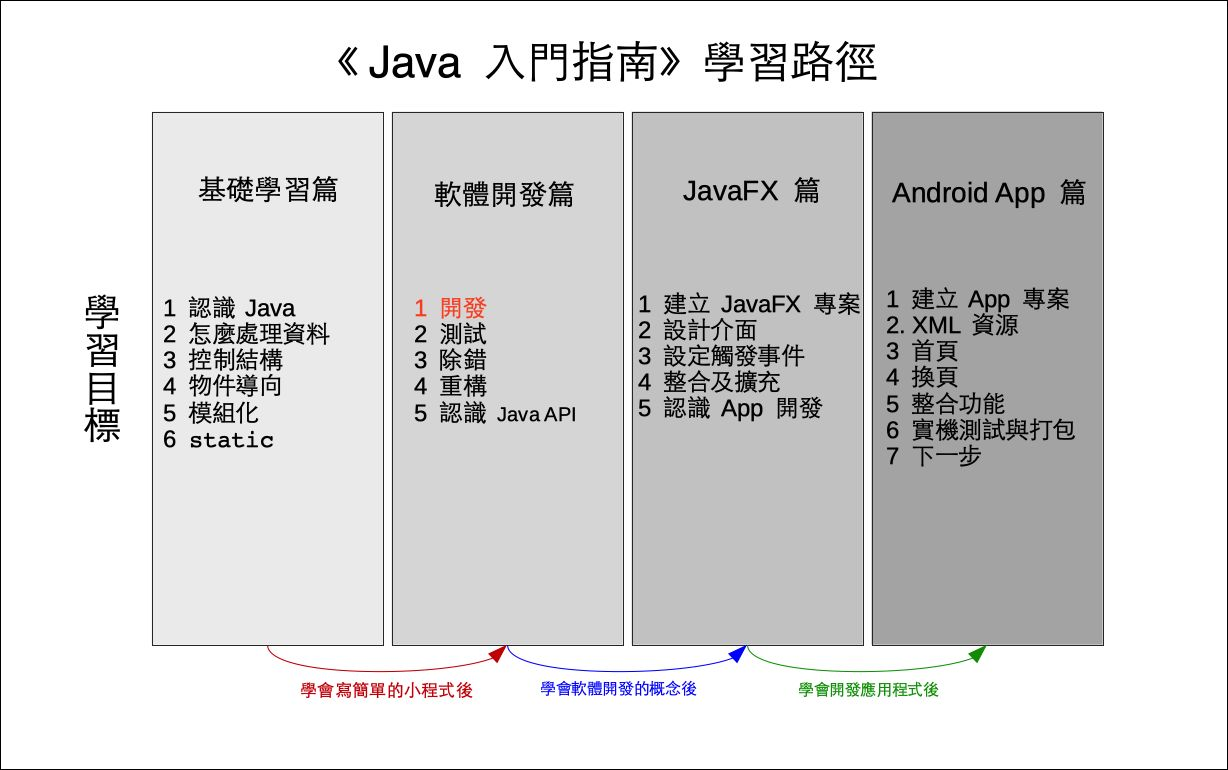# 單元 14 - 實作 setCode()

～～學習進度表～～setCode() 的工作就是建立密碼表，我們需要的密碼表就是一個攪亂順序的英文字母表

setCode()

 0 1 2 3 4 5 6 7 8 9 10 11 12 13 14 15 16 17 18 19 20 21 22 23 24 25 q z i r a j s b k t c l u d m v e n w f o x g p y h

```y = a * x + b
m = y % n
r = m + diff```

ab 預定是 09 之間的隨機整數，要取得隨機整數的話， API 有程式可用，倒是現在先不用急著一次到位，我們先把 a 設定成 3b 設定成 5 好了， setCode() 的實作如下

``````// setter
public void setCode() {
int a = 3;
int b = 5;

int x, y, m, i;
char c = 'a';
for (i = 0; i < 26; i++) {
x = c;
y = x * a + b;
m = y % 26;
code[i] = (char) (m + 97);
c++;
}
}``````

``````for (i = 0; i < 26; i++) {
x = c;
y = x * a + b;
m = y % 26;
code[i] = (char) (m + 97);
c++;
}``````

``x = c;``

``c++;``

Java 的字元型態其實就是 Unicode 編碼，因此可做算術運算。

``code[i] = (char) (m + 97);``

``````/*
* 檔名：Encrypt02.java
* 作者：張凱慶
* 網站：http://kaiching.org
*/
package encrypt02;

public class Encrypt02 {
// 密碼表字元陣列
private char[] code = new char;

// 建構子
public Encrypt02() {
setCode();
}

// setter
public void setCode() {
int a = 3;
int b = 5;

int x, y, m, i;
char c = 'a';
for (i = 0; i < 26; i++) {
x = c;
y = x * a + b;
m = y % 26;
code[i] = (char) (m + 97);
c++;
}
}

// getter
public char[] getCode() {
return code;
}

// 編碼的方法
public String toEncode(String s) {
return s;
}

// 解碼的方法
public String toDecode(String s) {
return s;
}

// 測試的 main()
public static void main(String[] args) {
Encrypt02 e = new Encrypt02();
System.out.println(e.getCode());
String s = "There is no spoon.";
System.out.println(s);
String s1 = e.toEncode(s);
System.out.println(s1);
String s2 = e.toDecode(s1);
System.out.println(s2);
}
}``````

 knqtwzcfiloruxadgjmpsvybeh There is no spoon. There is no spoon. There is no spoon.character字元
loop迴圈
variable變數
 1. The Java™ Tutorials: Declaring Classes 2. The Java™ Tutorials: Declaring Member Variables 3. The Java™ Tutorials: Defining Methods 4. The Java™ Tutorials: Providing Constructors for Your Classes 5. The Java™ Tutorials: Returning a Value from a Method 6. The Java™ Tutorials: Using the this Keyword 7. The Java™ Tutorials: Controlling Access to Members of a Class 8. The Java™ Tutorials: Understanding Class Members 9. The Java™ Tutorials: Initializing Fields

1. 實作 setCode() 是先將公式中的 a 設成 3b 設成 5 ，然後用迴圈利用兩個控制變數 ic 逐一計算每個字元。
2. 字元型態就是範圍較小的整數，因此可直接進行算術運算。

1. 為什麼不把建立對換表格直接寫在建構子裡就好了？
2. 為什麼要用小括弧圍住型態名稱，像是 (char)

1. 承接上一個單元的猜數字遊戲，另建立一個專案 Exercise1401 ，在 Exeercise1401 類別的 main() 方法接受使用者輸入，加入判斷使用者是否猜對的邏輯及提示訊息。
2. 承上題，另建立一個專案 Exercise1402 ，將 answer 改為字串。